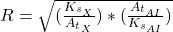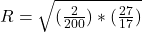## Consider the spacing of vibrational energy levels of materials X and Al based on the quantum harmonic oscillator model for interatomic bonds

Question

Consider the spacing of vibrational energy levels of materials X and Al based on the quantum harmonic oscillator model for interatomic bonds. X is a hypothetical material of stiffness ks = 2N/m and atomic mass 200 mN (where mN is the mass of a nucleon). The interatomic stiffness of Al is ks = 17N/m, and its atomic mass is 27 mN. What is the ratio of the energy level spacings, ∆EX ∆EAl ?

in progress 0
2 months 2021-07-28T07:16:55+00:00 1 Answers 0 views 0

The ratio is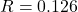Explanation:

From the question we are told that

The stiffness is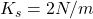The  atomic mass is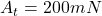The inter-atomic stiffness of Al is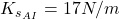The atomic mass of  AI is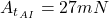The ratio of the energy is mathematically represented as